# Chemical Equilibrium Chapter 18 Equilibrium Few chemical reactions

• Slides: 23Chemical Equilibrium Chapter 18Equilibrium • Few chemical reactions proceed in only one direction – Most reactions are reversible N 2 + 3 H 2 2 NH 3 • At first the reaction proceeds towards the formation of product – As soon as some product is formed the reverse reaction begins (reactants are produced) N 2 + 3 H 2 ⇌ 2 NH 3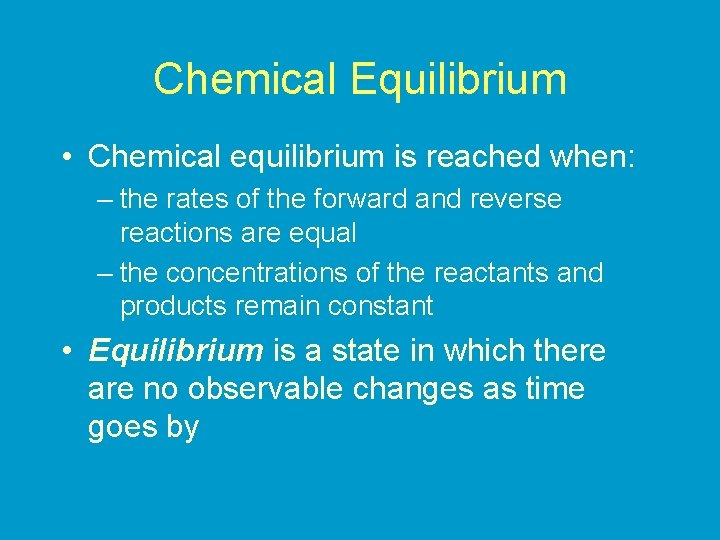Chemical Equilibrium • Chemical equilibrium is reached when: – the rates of the forward and reverse reactions are equal – the concentrations of the reactants and products remain constant • Equilibrium is a state in which there are no observable changes as time goes byH 2 O (l) ⇌ H 2 O (g) Physical equilibrium The changes that occur are physical processes colorless dark brown N 2 O 4 (g) ⇌ 2 NO 2 (g) Chemical equilibrium The changes that occur are chemicalN 2 O 4 (g) ⇌ 2 NO 2 (g) equilibrium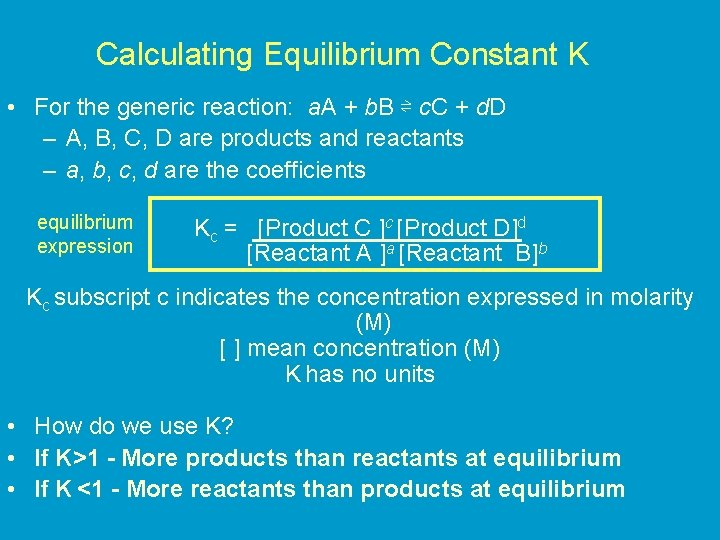Calculating Equilibrium Constant K • For the generic reaction: a. A + b. B ⇌ c. C + d. D – A, B, C, D are products and reactants – a, b, c, d are the coefficients equilibrium expression Kc = [Product C ]c [Product D]d [Reactant A ]a [Reactant B]b Kc subscript c indicates the concentration expressed in molarity (M) [ ] mean concentration (M) K has no units • How do we use K? • If K>1 - More products than reactants at equilibrium • If K <1 - More reactants than products at equilibriumN 2 O 4 (g) ⇌ 2 NO 2 (g) Equilibrium constant (Kc) 14. 1• Calculating Kc for a Homogenous Equilibrium In a homogenous equilibrium reactants and products are all in the same state (gas, liquid, solid): N 2(g) + 3 H 2(g) ⇌ 2 NH 3 (g) -all gases • For the above reaction write the equilibrium expression and calculate the equilibrium constant (Kc) using the following information: [NH 3] = 1. 866 M [N 2] = 1. 066 M [H 2] = 3. 200 M • Are there more products or reactants at equilibrium?Calculating KP • K can also be calculated using the partial pressures of the reactants and products (only works for gaseous reactions) • This is because at a constant temperature pressure of a gas is directly related to its concentration in mol/L: PV = n. RT P= n. RT V a. A(g) ⇌ b. B(g) K P = P Bb P AaCalculating KP a. A(g) ⇌ b. B(g) K P = P Bb P Aa N 2 O 4(g) ⇌ 2 NO 2(g) KP = PNO 2 PN O 2 2 4Kc and Kp • In general Kc and Kp are not equal • The relationship between Kc and Kp: Kp = Kc(RT)∆n or Kp = Kc(0. 0821 T)∆n ∆n = moles of gaseous products – moles of gaseous = (c + d) – (a + b) coeff. reactants or ∑ product coeff. – ∑ reactant • when ∆n = 0, Kp = Kc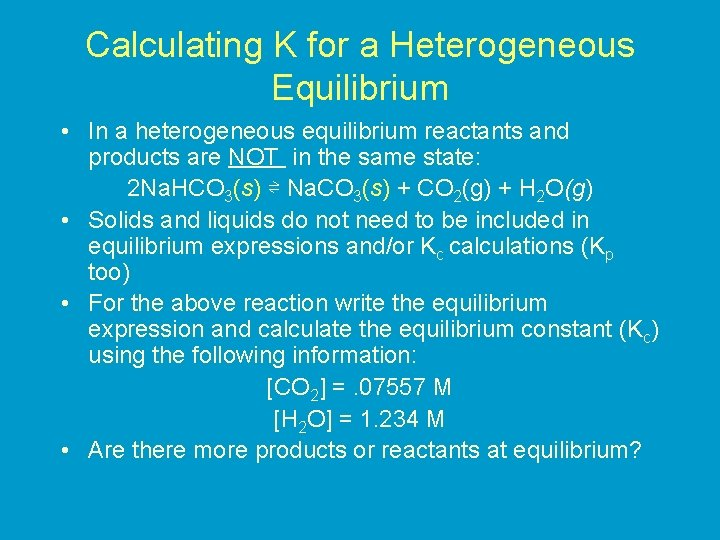Calculating K for a Heterogeneous Equilibrium • In a heterogeneous equilibrium reactants and products are NOT in the same state: 2 Na. HCO 3(s) ⇌ Na. CO 3(s) + CO 2(g) + H 2 O(g) • Solids and liquids do not need to be included in equilibrium expressions and/or Kc calculations (Kp too) • For the above reaction write the equilibrium expression and calculate the equilibrium constant (Kc) using the following information: [CO 2] =. 07557 M [H 2 O] = 1. 234 M • Are there more products or reactants at equilibrium?Practice 1. 2. 1. The equilibrium concentrations for the reaction between carbon monoxide and molecular chlorine to form COCl 2 (g) at 740 C are [CO] = 0. 012 M, [Cl 2] = 0. 054 M, and [COCl 2] = 0. 14 M. Calculate the equilibrium constants Kc and Kp. The equilibrium constant Kp for the following reaction is 158 at 1000 K. What is the equilibrium pressure of O 2 if the PNO 2 = 0. 400 atm and PNO = 0. 270 atm 2 NO 2 (g) ⇌ 2 NO (g) + O 2 (g) Consider the equilibrium below at 295 K. The partial pressure of each gas is 0. 265 atm. Calculate Kp and Kc for the reaction. NH 4 HS (s) ⇌ NH 3 (g) + H 2 S (g)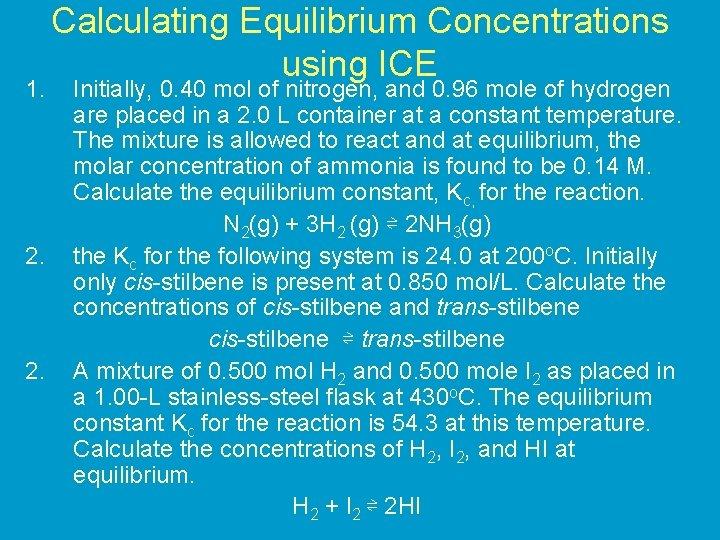1. 2. Calculating Equilibrium Concentrations using ICE Initially, 0. 40 mol of nitrogen, and 0. 96 mole of hydrogen are placed in a 2. 0 L container at a constant temperature. The mixture is allowed to react and at equilibrium, the molar concentration of ammonia is found to be 0. 14 M. Calculate the equilibrium constant, Kc, for the reaction. N 2(g) + 3 H 2 (g) ⇌ 2 NH 3(g) the Kc for the following system is 24. 0 at 200 o. C. Initially only cis-stilbene is present at 0. 850 mol/L. Calculate the concentrations of cis-stilbene and trans-stilbene cis-stilbene ⇌ trans-stilbene A mixture of 0. 500 mol H 2 and 0. 500 mole I 2 as placed in a 1. 00 -L stainless-steel flask at 430 o. C. The equilibrium constant Kc for the reaction is 54. 3 at this temperature. Calculate the concentrations of H 2, I 2, and HI at equilibrium. H 2 + I 2 ⇌ 2 HI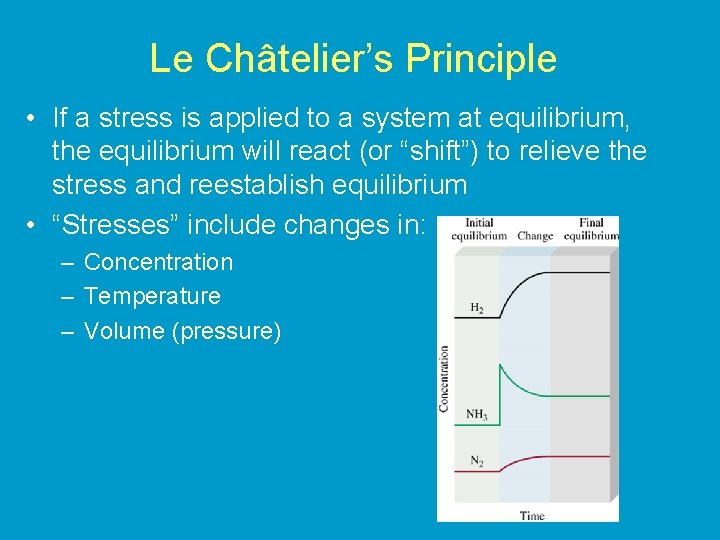Le Châtelier’s Principle • If a stress is applied to a system at equilibrium, the equilibrium will react (or “shift”) to relieve the stress and reestablish equilibrium • “Stresses” include changes in: – Concentration – Temperature – Volume (pressure)Changes in Concentration • If product or reactant is added to a system at equilibrium the system will react by shifting in the direction opposite the addition • If product or reactant is removed the system shifts in the direction of the removal – The system reacts to restore the concentration of the substance that is removed Change Shift in equilibrium Increase concentration of reactant Shift to the right (towards products) Increase concentration of product Shift to the left (towards reactants) Remove reactant Shift to the left Remove product Shift to the right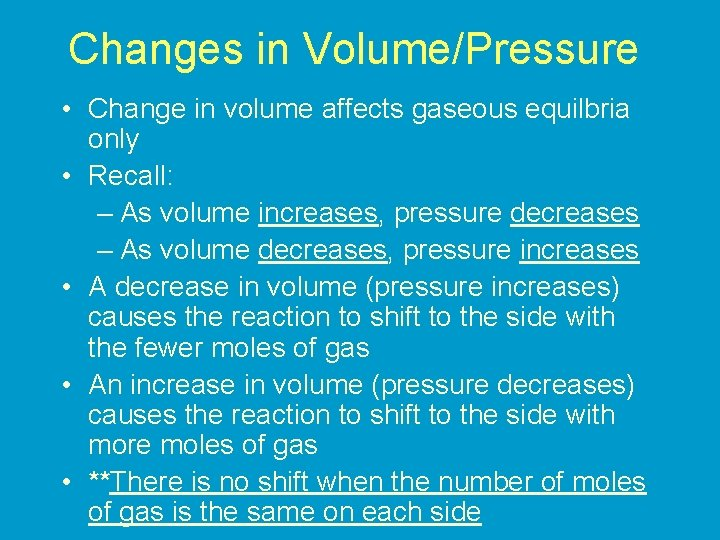Changes in Volume/Pressure • Change in volume affects gaseous equilbria only • Recall: – As volume increases, pressure decreases – As volume decreases, pressure increases • A decrease in volume (pressure increases) causes the reaction to shift to the side with the fewer moles of gas • An increase in volume (pressure decreases) causes the reaction to shift to the side with more moles of gas • **There is no shift when the number of moles of gas is the same on each side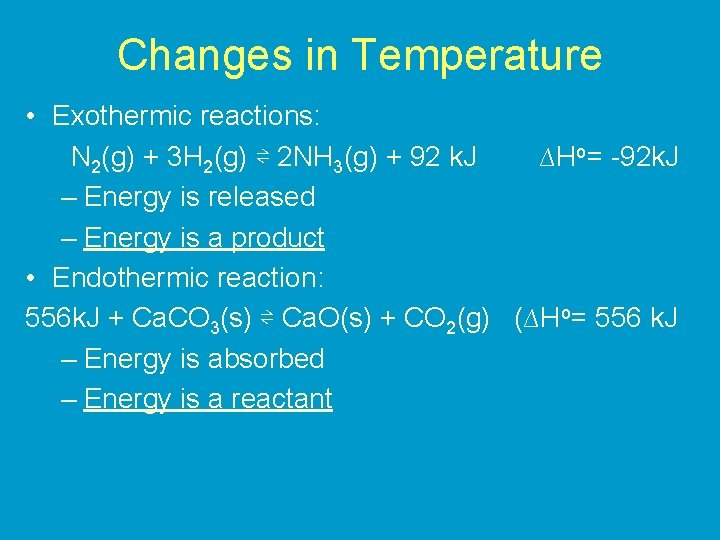Changes in Temperature • Exothermic reactions: N 2(g) + 3 H 2(g) ⇌ 2 NH 3(g) + 92 k. J ∆Ho= -92 k. J – Energy is released – Energy is a product • Endothermic reaction: 556 k. J + Ca. CO 3(s) ⇌ Ca. O(s) + CO 2(g) (∆Ho= 556 k. J – Energy is absorbed – Energy is a reactantChanges in Temperature • Treat energy as a reactant/product-Rules for changes in concentration apply • Increasing the temperature: Energy is being added – the system will react by shifting in the direction opposite the addition – equilibrium shifts in the direction that uses up energy (heat) • Decreasing temperature: Energy is being removed – System to shifts restore the energy lost – equilibrium shifts in the direction that creates energy (heat)Changes in Temperature • Value of K changes with changes in temperature – (K does not change with changes in pressure or concentration--only position of equilibrium changes) • Exothermic reactions (energy is a product) – K decreases when temperature increases • Endothermic reactions (energy is a reactant) – K increases when temperature is increasedAddition of Catalyst • Adding a Catalyst • does not change K • does not shift the position of an equilibrium system • system will reach equilibrium sooner uncatalyzedLe Châtelier’s Principle in summary Change Shift Equilibrium Change Equilibrium Constant Concentration yes no Pressure yes no Volume yes no Temperature yes Catalyst no no